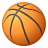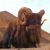Announcements
You can succeed best and quickest by helping others to succeed. Join the conversation.
cancel
Showing results for
Did you mean:Contributor III

## Rank Top 10 for a month

Hi,

I just about understand the rank function but believe I need to also introduce SET analysis into the mix also.

I have a formula =sum(if(CIC_COMPARABLE = 'Plan', CIC_NETINCOME,0))

I also have a field CIC_INCOME_REC_MONTH

My goal is to simply show the Top 10 'Plan' for the Current Month - whatever month we are in.

Would any be able to help me on this please.

Thanks,

Rob

1 Solution

Accepted SolutionsContributor III

Try this:

Sum({<CIC_COMPARABLE = {'Plan'} ,CIC_INCOME_RECOGNITION_MONTH = {"=Month(today())"}, CIC_DEBTORCODE = {"=Rank(sum(CIC_NETINCOME))<=10"}>} CIC_NETINCOME)

Make sure both month formats in source and output are same. output format will be like Jan, Nov....

10 RepliesSpecialist

Try this:

sum( {<CIC_COMPARABLE = 'Plan', CIC_INCOME_REC_MONTH = {"\$(=Month(Today())"}>}CIC_NETINCOME)

(if CIC_INCOME_REC_MONTH  is a date)Creator III

Try This

Dimension

=If(Aggr(Rank(Sum(CIC_COMPARABLE)), [CIC_NETINCOME])<=10, [ IC_NETINCOME], 'Others')Contributor III

Hi Rob,

Top 10(Customers,products etc..) has to be based on some value which may be sales.

Try this:

Sum({<CIC_Comparable ={"Plan"},CIC_Income_rec_Month={"\$(=Month(Today())"},Customer = {"=Rank(sum(Sales))<=10"}>} Sales)Contributor III
Author

Hi Afroz,

sum( {<CIC_COMPARABLE = {'Plan'} , CIC_INCOME_RECOGNITION_MONTH = {"\$(=month(today()))"}>} CIC_NETINCOME).

Thanks for very prompt answer I had to enclose Plan with {} to make work.

Thanks,

RobContributor III

Does your expression give you Top 10 records?Contributor III
Author

Hi Afroz,

I put presentation to 10 lines.... not the best idea.

Below is trying tp put actual field names in based your working - no error's in formula but not no results.

Sum({<CIC_COMPARABLE = {"Plan"} , CIC_INCOME_RECOGNITION_MONTH = {"\$(=Month(Today())"}, CIC_DEBTORCODE = {"=Rank(sum(CIC_NETINCOME))<=10"}>} CIC_NETINCOME)

Any thoughts

Thanks,

RobContributor III

Try this:

Sum({<CIC_COMPARABLE = {'Plan'} ,CIC_INCOME_RECOGNITION_MONTH = {"=Month(today())"}, CIC_DEBTORCODE = {"=Rank(sum(CIC_NETINCOME))<=10"}>} CIC_NETINCOME)

Make sure both month formats in source and output are same. output format will be like Jan, Nov....Contributor III
Author

Spot on thank you - really appreciate your time on thisContributor III
Author

Hi Afroz,

I thought it was working but it afroz.shaik‌ but the Rank piece appears not to be looking at just the month but rather the whole year.Our new formula is supposed be the same as the Plan values

Plan  is

sum( {<CIC_COMPARABLE = {'Plan'} , CIC_INCOME_RECOGNITION_MONTH = {"\$(=month(today()))"}>} CIC_NETINCOME)

New formula is

Sum({<CIC_COMPARABLE = {'Plan'} , CIC_INCOME_RECOGNITION_MONTH = {"\$(=month(today()))"}, CIC_DEBTORCODE = {"=Rank(sum(CIC_NETINCOME))<=10"}>} CIC_NETINCOME)

Does the Rank element need to also include month ? I did try and kept getting errors ( or blanks ) - I'd appreciate anything you can do on this.

Thanks for your time on this.

RobCommunity Browser